## 5.1.1 Modeling Droplet Atomization

The atomizing nozzle from a spray pyrolysis process can be simulated using random number distributions for the generated size of the droplet, the initial droplet velocity, and the droplet direction as it leaves the atomizer. The radius of a droplet, formed from a liquid which is forced through a thin circular outlet is given by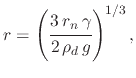(160)

where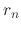is the outlet radius,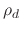is the liquid density, and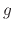is the gravitational constant. The variable which can be modified in order to decrease the radius of the droplet is the circular outlet radius. This radius, however, has fabrication limitations so a better method to decrease the droplet radius is to increase the force acting on the liquid as it is passing through the outlet. Equation (5.1) assumes that only gravity is acting towards this goal, but providing an additional push'' can lead to a significant reduction in the size of the droplet. The influence of an additional aerodynamic drag force is summarized with the critical Weber number: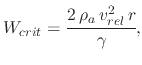(161)

where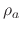is the air gas density,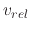is the relative velocity. The critical Weber number has a value of 22 for a free falling droplet and 13 for a droplet which is suddenly exposed to a high velocity. Therefore, a sudden increase in a droplet's velocityis counter-acted with a decrease in the droplet radius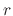. The large droplets are broken up into much smaller components, useful for spray pyrolysis. This additional force is provided using air pressure in the case of a PSD system, and an electric field in the case of an ESD system. The generation of droplets can easily be modeled using the given equations; however, most atomizer manufacturers directly provide the droplet diameter distributions under various pressure conditions. If all information required for (5.1) and (5.2) is known, a model can be implemented to use this information; however, if the atomizer manufacturer's data is available instead, then the provided information should be used in the atomization model.

The next requirement from the atomizer is the charging of the droplet. Each droplet contains charged particles from the precursor solution which will form the thin film, deposited on the wafer surface. The maximum charge for a droplet is given by the Rayleigh limit (4.6). The average charge over volume for droplets calculated by Larriba-Andaluz and Fernandez de la Mora  is shown to be approximately 58% of the Rayleigh limit, regardless of the liquid flow rate. This value is used in the model to calculate the effects of the electrical force on each droplet as well as the amount of solvent available for the deposition process.

Hendricks  measured the droplet diameter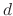's relationship with its charge and showed that a relation between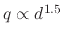and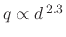exists using an electrospray which produces a polydisperse droplet size distribution between 0.1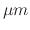and 10. More recently, Wilhelm  showed that the relationship for a cone-jet ESD spray is, while for a multi-jet electrospray system a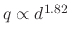is more appropriate. The presence of charges in a droplet assumes the presence of a solvent ion, which is to deposit on the wafer surface. Therefore, the amount of charge will be the deciding factor in the amount of deposited material each droplet will contribute to the film growth.

L. Filipovic: Topography Simulation of Novel Processing Techniques